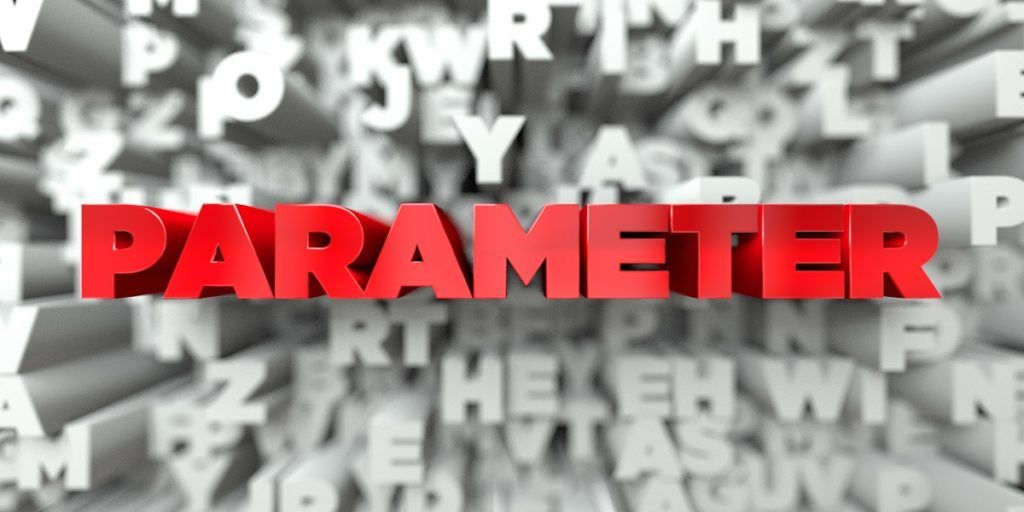# Parameter

Characteristics that are used to define a given population

## What is Parameter?

A parameter is a useful component of statistical analysis. It refers to the characteristics that are used to define a given population. It is used to describe a specific characteristic of the entire population. When making an inference about the population, the parameter is unknown because it would be impossible to collect information from every member of the population. Rather, we use a statistic of a sample picked from the population to derive a conclusion about the parameter.For example, the parameter can be used to describe the mean amount of loans that are awarded to the students of ABC University. Assuming that the population of the university is 3,000, the researcher can start by calculating the financial aid of a few select samples of the population, or about 10 students. For three samples of 10 students each, the researcher may obtain a mean of \$2,000, \$1,200, and \$800. The researcher can use this sample mean to make an inference about the population parameter.

### Most Common Parameters

The most commonly used parameters are the measures of central tendency. The measures include mean, median and mode, and they are used to describe how data behaves in a distribution. They are discussed below:

#### 1. Mean

The mean is also referred to as the average, and it is the most commonly used among the three measures of central tendency. Researchers use the parameter to describe the data distribution of ratios and intervals.

The mean is obtained by summing and dividing the values by the number of scores. For example, in five households that comprise 5, 2, 1, 3 and 2 children, the mean can be calculated as follows:

= (5+2+1+3+2)/5

= 13/5

= 2.6

#### 2. Median

The median is used to calculate variables that are measured with ordinal, interval or ratio scales. It is obtained by arranging the data from the lowest to the highest and then picking the number (s) at the middle. If the total number of data points is an odd number, the median is usually the middle number. If the numbers are even, the median is obtained by summing the two numbers at the middle and dividing them by two to get the mean.

Median is mostly used when there are a few data points that are different. For example, when calculating the median of students entering college, there may be a section of students who are older than the rest. Using the mean may distort the values since it will show that the average age of students entering college to be very high, whereas using median can give a true reflection of the situation.

For example, let’s find the median age of student entering college for the first time, given the following values of ten students:

17, 17, 18, 19, 19, 20, 21, 25, 28, 32

The median of the values above is (19+20)/2 = 19.5.

#### Mode

The mode is the most occurring number within a data distribution. It shows what number or value is the highest in number or most common in the data distribution. The mode is used for any type of data.

For example, let’s take the example of a college class with about 40 students. The students are given a test exam, graded, and then grouped on a scale of 1-5, starting with students with the lowest number of marks.

The marks are graded as follows:

• Cluster 1: 5
• Cluster 2: 7
• Cluster 3: 13
• Cluster 4: 12
• Cluster 5: 3

Cluster 3 shows the highest number of students, and therefore, the mode is 13. It demonstrates that out of 40 students, most of the students were graded in cluster 3.

### Parameter and Statistics

A parameter is used to describe the entire population being studied. For example, we want to know the average length of a butterfly. It is a parameter because it is states something about the entire population of butterflies.

Parameters are difficult to obtain, but we use the corresponding statistic to estimate its value. A statistic describes a sample of a population, while a parameter describes the entire population. Since it will be impossible to catch and measure all the butterflies in the world, we can catch 100 butterflies and measure their length. The mean length of the 100 butterflies is a statistic that we can use to make an inference about the length of the entire butterfly population.

Typically, the value of a statistic can vary from one sample to another, while the parameter remains fixed. For example, one sample of 100 butterflies may have an average length of 6.5 mm, while another sample of 100 butterflies from another region may have an average length of 6.8 mm.

Also, a smaller sample of 50 butterflies may have an average length of 7.0 mm. The statistic obtained from the sample of the population can then be used to estimate the parameter of the entire population.

### More Resources

CFI is the official provider of the Financial Modeling and Valuation Analyst (FMVA)™ certification program, designed to transform anyone into a world-class financial analyst.

To keep learning and developing your knowledge of financial analysis, we highly recommend the additional resources below:

• Hypothesis Testing
• Nonparametric Tests
• Quantitative Analysis
• Sample Selection Bias

### Financial Analyst Certification

Become a certified Financial Modeling and Valuation Analyst (FMVA)® by completing CFI’s online financial modeling classes and training program!